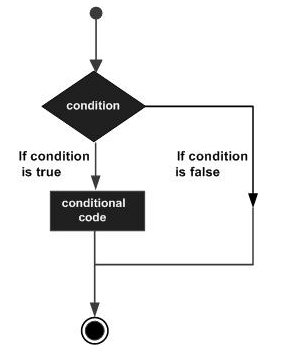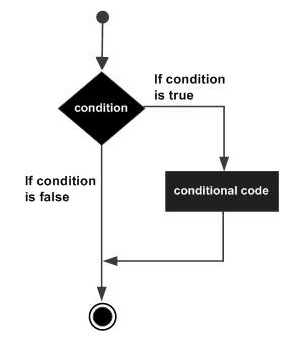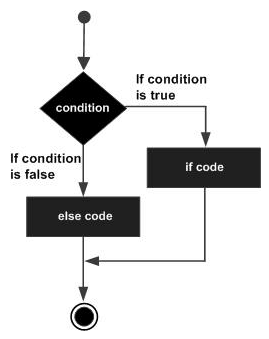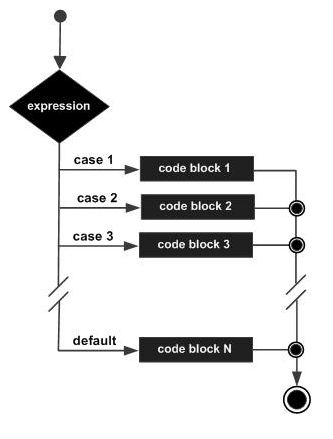Basic Decision Making in C Programming

# Basic Decision Making in C Programming - GATE Computer Science Engineering(CSE) 2023 Mock Test Series - Computer Science Engineering (CSE)

Decision making structures require that the programmer specifies one or more conditions to be evaluated or tested by the program, along with a statement or statements to be executed if the condition is determined to be true, and optionally, other statements to be executed if the condition is determined to be false.

Show below is the general form of a typical decision making structure found in most of the programming languages −C programming language assumes any non-zero and non-null values as true, and if it is either zero or null, then it is assumed as false value.

C programming language provides the following types of decision making statements.

 S.N. Statement & Description 1 if statmentAn if statement consists of a boolean expression followed by one or more statements. 2 if...else statmentAn if statement can be followed by an optional else statement, which executes when the Boolean expression is false. 3 nested if statmentsYou can use one if or else if statement inside another if or else if statement(s). 4 switch statmentA switch statement allows a variable to be tested for equality against a list of values. 5 nested switch statment You can use one switch statement inside another switch statement(s).

## The ? : Operator

We have covered conditional operator ? : in the previous chapter which can be used to replace if...else statements. It has the following general form −

`Exp1 ? Exp2 : Exp3;`

Where Exp1, Exp2, and Exp3 are expressions. Notice the use and placement of the colon.

The value of a ? expression is determined like this −

• Exp1 is evaluated. If it is true, then Exp2 is evaluated and becomes the value of the entire ? expression.

• If Exp1 is false, then Exp3 is evaluated and its value becomes the value of the expression.

## if statement

An if statement consists of a Boolean expression followed by one or more statements.

## Syntax

The syntax of an 'if' statement in C programming language is −

`if(boolean_expression) {   /* statement(s) will execute if the boolean expression is true */}`

If the Boolean expression evaluates to true, then the block of code inside the 'if' statement will be executed. If the Boolean expression evaluates to false, then the first set of code after the end of the 'if' statement (after the closing curly brace) will be executed.

C programming language assumes any non-zero and non-null values as true and if it is either zero or null, then it is assumed as false value.

## Flow Diagram## Example

`#include <stdio.h> int main () {   /* local variable definition */   int a = 10;    /* check the boolean condition using if statement */	   if( a < 20 ) {      /* if condition is true then print the following */      printf("a is less than 20" );   }      printf("value of a is : %d", a);    return 0;}`

When the above code is compiled and executed, it produces the following result −

`a is less than 20;value of a is : 10`

if...else statement

An if statement can be followed by an optional else statement, which executes when the Boolean expression is false.

## Syntax

The syntax of an if...else statement in C programming language is −

`if(boolean_expression) {   /* statement(s) will execute if the boolean expression is true */}else {   /* statement(s) will execute if the boolean expression is false */}`

If the Boolean expression evaluates to true, then the if block will be executed, otherwise, the else block will be executed.

C programming language assumes any non-zero and non-null values as true, and if it is either zero or null, then it is assumed as false value.

## Flow Diagram## Example

`#include <stdio.h> int main () {   /* local variable definition */   int a = 100;    /* check the boolean condition */   if( a < 20 ) {      /* if condition is true then print the following */      printf("a is less than 20" );   }   else {      /* if condition is false then print the following */      printf("a is not less than 20" );   }      printf("value of a is : %d", a);    return 0;}`

When the above code is compiled and executed, it produces the following result −

`a is not less than 20;value of a is : 100`

## If...else if...else Statement

An if statement can be followed by an optional else if...else statement, which is very useful to test various conditions using single if...else if statement.

When using if...else if..else statements, there are few points to keep in mind −

• An if can have zero or one else's and it must come after any else if's.

• An if can have zero to many else if's and they must come before the else.

• Once an else if succeeds, none of the remaining else if's or else's will be tested.

### Syntax

The syntax of an if...else if...else statement in C programming language is −

`if(boolean_expression 1) {   /* Executes when the boolean expression 1 is true */}else if( boolean_expression 2) {   /* Executes when the boolean expression 2 is true */}else if( boolean_expression 3) {   /* Executes when the boolean expression 3 is true */}else  {   /* executes when the none of the above condition is true */}`

Example

`#include <stdio.h> int main () {   /* local variable definition */   int a = 100;    /* check the boolean condition */   if( a == 10 ) {      /* if condition is true then print the following */      printf("Value of a is 10" );   }   else if( a == 20 ) {      /* if else if condition is true */      printf("Value of a is 20" );   }   else if( a == 30 ) {      /* if else if condition is true  */      printf("Value of a is 30" );   }   else {      /* if none of the conditions is true */      printf("None of the values is matching" );   }      printf("Exact value of a is: %d", a );    return 0;}`

When the above code is compiled and executed, it produces the following result −

`None of the values is matchingExact value of a is: 100`

nested if statements

It is always legal in C programming to nest if-else statements, which means you can use one if or else if statement inside another if or else if statement(s).

## Syntax

The syntax for a nested if statement is as follows −

`if( boolean_expression 1) {   /* Executes when the boolean expression 1 is true */   if(boolean_expression 2) {      /* Executes when the boolean expression 2 is true */   }}`

You can nest else if...else in the similar way as you have nested if statements.

## Example

`include <stdio.h> int main () {   /* local variable definition */   int a = 100;   int b = 200;    /* check the boolean condition */   if( a == 100 ) {         /* if condition is true then check the following */      if( b == 200 ) {         /* if condition is true then print the following */         printf("Value of a is 100 and b is 200" );      }   }      printf("Exact value of a is : %d", a );   printf("Exact value of b is : %d", b );    return 0;}`

When the above code is compiled and executed, it produces the following result −

`Value of a is 100 and b is 200Exact value of a is : 100Exact value of b is : 200`

## switch statement

switch statement allows a variable to be tested for equality against a list of values. Each value is called a case, and the variable being switched on is checked for each switch case.

## Syntax

The syntax for a switch statement in C programming language is as follows −

`switch(expression) {   case constant-expression  :      statement(s);      break; /* optional */	   case constant-expression  :      statement(s);      break; /* optional */     /* you can have any number of case statements */   default : /* Optional */   statement(s);}`

The following rules apply to a switch statement −

• The expression used in a switch statement must have an integral or enumerated type, or be of a class type in which the class has a single conversion function to an integral or enumerated type.

• You can have any number of case statements within a switch. Each case is followed by the value to be compared to and a colon.

• The constant-expression for a case must be the same data type as the variable in the switch, and it must be a constant or a literal.

• When the variable being switched on is equal to a case, the statements following that case will execute until a break statement is reached.

• When a break statement is reached, the switch terminates, and the flow of control jumps to the next line following the switch statement.

• Not every case needs to contain a break. If no break appears, the flow of control will fall through to subsequent cases until a break is reached.

• switch statement can have an optional default case, which must appear at the end of the switch. The default case can be used for performing a task when none of the cases is true. No break is needed in the default case.

## Flow Diagram## Example

`#include <stdio.h> int main () {   /* local variable definition */   char grade = 'B';   switch(grade) {      case 'A' :         printf("Excellent!" );         break;      case 'B' :      case 'C' :         printf("Well done" );         break;      case 'D' :         printf("You passed" );         break;      case 'F' :         printf("Better try again" );         break;      default :         printf("Invalid grade" );   }      printf("Your grade is  %c", grade );    return 0;}`

When the above code is compiled and executed, it produces the following result −

`Well doneYour grade is B`
The document Basic Decision Making in C Programming | GATE Computer Science Engineering(CSE) 2023 Mock Test Series - Computer Science Engineering (CSE) is a part of the Computer Science Engineering (CSE) Course GATE Computer Science Engineering(CSE) 2023 Mock Test Series.
All you need of Computer Science Engineering (CSE) at this link: Computer Science Engineering (CSE)

## FAQs on Basic Decision Making in C Programming - GATE Computer Science Engineering(CSE) 2023 Mock Test Series - Computer Science Engineering (CSE)

 1. What is decision making in C programming?Ans. Decision making in C programming refers to the process of making choices or taking actions based on certain conditions or criteria. It involves using conditional statements like if-else, switch-case, and loops to control the flow of execution in a program based on different conditions.
 2. What are the different types of decision-making statements in C programming?Ans. In C programming, there are primarily three types of decision-making statements: a) if-else statement: It allows the program to make a decision based on a condition. If the condition is true, the code inside the if block is executed, otherwise, the code inside the else block is executed. b) switch-case statement: It allows the program to select one of many code blocks to be executed based on the value of an expression. c) loop statements: While loop, do-while loop, and for loop are used for repetitive decision making in C programming.
 3. How do you write an if-else statement in C programming?Ans. The if-else statement in C programming is written as follows: if (condition) { // code to be executed if the condition is true } else { // code to be executed if the condition is false } The condition is an expression that evaluates to either true or false. If the condition is true, the code inside the if block is executed. If the condition is false, the code inside the else block is executed.
 4. What is the purpose of a switch-case statement in C programming?Ans. The switch-case statement in C programming is used when we have multiple options and we need to perform different actions based on the value of a variable or an expression. It allows the program to select one of many code blocks to be executed based on the value of the expression. The syntax of the switch-case statement is as follows: switch (expression) { case constant1: // code to be executed if expression matches constant1 break; case constant2: // code to be executed if expression matches constant2 break; ... default: // code to be executed if expression doesn't match any constant break; } The expression is evaluated, and if it matches any of the constants, the corresponding code block is executed. If no match is found, the code inside the default block is executed.
 5. How do you write a for loop in C programming for decision making?Ans. The for loop in C programming is commonly used for decision making based on a condition. It is written as follows: for (initialization; condition; increment/decrement) { // code to be executed repeatedly as long as the condition is true } The initialization part is executed only once before the loop starts. The condition is evaluated before each iteration, and if it is true, the code inside the loop is executed. After each iteration, the increment/decrement part is executed. If the condition becomes false, the loop is terminated, and the program continues executing the code after the loop.

## GATE Computer Science Engineering(CSE) 2023 Mock Test Series

151 docs|215 tests

## GATE Computer Science Engineering(CSE) 2023 Mock Test Series

151 docs|215 tests
Signup to see your scores go up within 7 days! Learn & Practice with 1000+ FREE Notes, Videos & Tests.
10M+ students study on EduRev
Track your progress, build streaks, highlight & save important lessons and more!(Scan QR code)
Related Searches

,

,

,

,

,

,

,

,

,

,

,

,

,

,

,

,

,

,

,

,

,

;